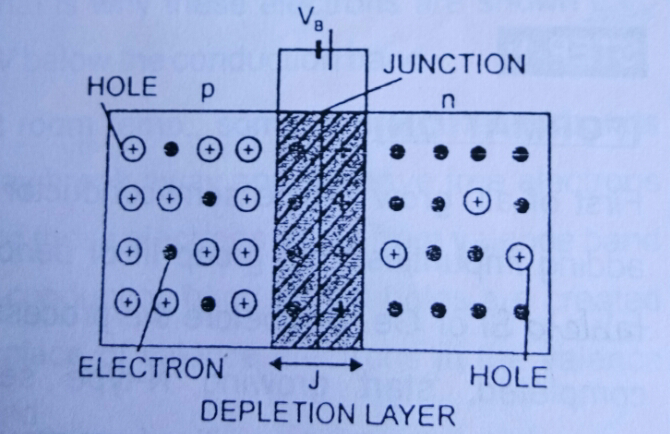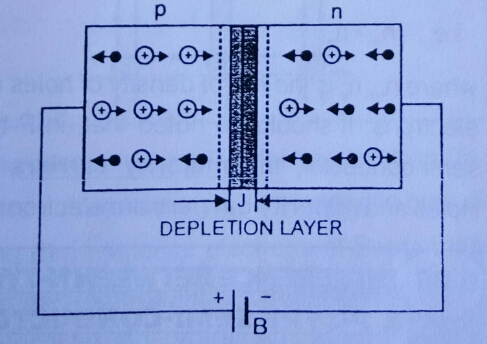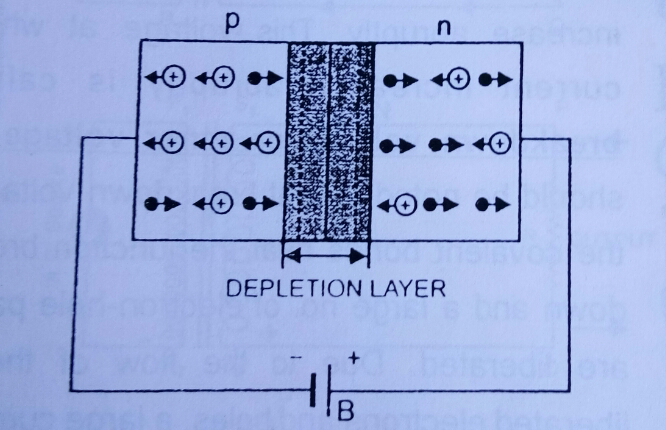# PN Junction diode - PN Junction

Views

## PN Junction diode

The concept of PN junction plays an important role in the field of semi-conductors. This concept finds many uses in all manner of electronic circuit designs. This concept really brought a revolutionary change in electronic physics.Here in this article I had covered  topics like ,p n junction definition ,formation , properties and types of p n junction.

What is PN Junction ?

When a P-type semi-conductor is brought in close contact with N-type semiconductor, a P-N junction is obtained. The P-N junction is also called as junction Diode.

Formation of P-N Junction :

First what we have to do is to start growing p-type semi-conductor by adding impurities from the group third of the periodic table. Before completing this process start growing n-type semi-conductor by adding impurities from the group fifth of the periodic table. Thus the crystal obtained is one single whole, One half of which functions as p-type and other half of which functions as n-type. There is a well defined junction between the two portions.

PN Junction diode

What is potential barrier ?

You may be thinking that why there is a potential barrier between the junction. Let me clarify that the difference in the  concentration of holes and electrons give rise to this barrier.

The electrons from N-region diffuse through the junction into p-region and the holes from p-region diffuse through the junction into the N-region.

The accumulation of electric charges of opposite polarities in the two regions of the junction give rise to electric field. This is as if a fictitious battery is connected across the junction. This electric field further opposes the flow of electrons from N-region to p-region and also opposes the flow of holes from p-region to N-region. Hence ,this electric field  sets as a potential barrier VB at the junction. This potential barrier opposes further diffusion of electrons and holes in opposite regions.PN Junction
The potential barrier for Ge is 0.3 volts and 0.7volts for silicon.

What is Depletion Layer ?

This layer is actually present on both sides of junction and carries no charge.

Types of Biasing :

There are actually two types of biasing namely forward biasing and reverse biasing :

a) Forward Biasing :

When we connect the positive terminal of the  external battery B to the p-side and the negative terminal of external battery B to the n-side then we get a P-N junction which is forward biased.PN Junction
If the voltage applied by the battery is greater than potential barrier, then the holes in p-region will cross the potential barrier and enter the N-region and the electrons will also cross the potential barrier and reach the p-region. Hence electrons and holes both start flowing in opposite directions and hence they constitute the flow of current in the semi-conductor . In this forward biasing, both potential barrier and depletion layer are reduced.

Thus we can also say, it offers low resistance to the flow of current in the case of forward biasing.

2) Reverse Biasing :

A P-N junction is said to be reverse biased, if the positive terminal of external battery is connected to n-side and negative terminal is connected to the p-side of the P-N junction.PN Junction
In this case, the majority carriers in the two regions are pulled away from the junction and hence there is no flow of majority carriers across the junction. Hence, no forward current flows through the junction, when it is reverse biased. In this case, both potential barrier and depletion layer become thick.

At last we can say that P-N junction offers high resistance to the forward current in reverse biasing.

PN Junction diode

Properties of P-N junction:

The P-N junction is having some  essential properties for modern electronics. A p-doped semiconductor is conductive . The same thing  is true for  an n-doped semiconductor, but the junction which is  between them can become depleted of carriers , and hence non-conductive, depending on the relative voltages of the two semiconductor regions. By manipulating this non conductive region, P-N junction can be used as diodes means circuit elements which offer the flow of current in One Direction but not in the opposite direction.

As we know bias is the application of a voltage across P-N junction . Both forward and reverse bias imply that it can be used as a diode. A P-N junction diode allows flow of charge carriers in One Direction but not in the opposite direction ,that means negative charges (electrons)  can only flow from N to P but not from P to N and positive charge (holes) can flow from P to N but not from N to P.

Characteristics of P-N junction diode :

P-N junction  diode basically have two characteristics that's forward characteristics and reverse characteristics.

Forward characteristics :

Take a P-N junction diode, a milliammeter and potential dividing arrangement and connect them in series. Also connect voltmeter in parallel with P-N junction.

It should be noted that the potential dividing arrangement should be connected in such a way such that the P-N junction should be forward biased.

Now, go on increasing the forward biased voltage and at each time, note down the corresponding value of forward current. After taking several readings, now plot the graph between forward biased voltage and current. We will get the curve ,which will represent the forward characteristics of P-N junction.

Reverse characteristics :

The graph between the reverse biased voltage and the corresponding reverse current across the P-N junction is known as reverse characteristics.

Take a P-N junction , a milliammeter, potential dividing arrangement and connect them in series with P-N junction. Also connect the voltmeter in parallel with P-N junction.
It should be noted that the potential dividing arrangement is to  connect in such a way that the P-N junction is reverse biased.

Now, go on increasing the reverse biased voltage applied to P-N junction and note down the corresponding reverse current. After taking several readings , plot the graph for  the values of reverse current and reverse biased voltage. We will now get the curve which will represent the reverse characteristics of P-N junction.

Some definitions to remember;

Knee Voltage :
The voltage at which or above which current starts increasing rapidly is called knee voltage.

Breakdown  Voltage  or Zener  voltage :

The voltage at which current increases abruptly is called the breakdown voltage or Zener Voltage. It should be noted that at breakdown voltage large current flows through the P-N junction due to the breakdown of covalent bonds near the junction.

https://www.themegaguide.com/

This article covers the definitions related to the p n junction including its properties and types . This article further provides you information  about the forward and reverse characteristics of the p n junction . Hope you enjoy this and comment me in the comment box about it.

1.